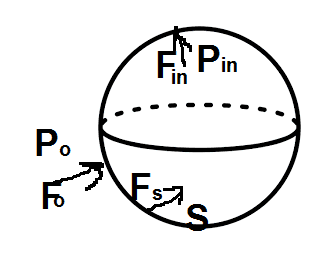# Pressure inside a water drop

• Pushoam
That is, the force due to outside pressure on one hemisphere, and the force due to surface tension on the other hemisphere.2) force ## F_{in}## in radially outward direction due to the inside pressure ##P_{in}##For that to make sense, you need to know the magnitude of the force due to outside pressure.3) force ##F_s## in radially inward direction due to the surface tension SFor that to make sense, you need to know the magnitude of the force due to surface tension.f

#### Pushoam

Homework Statement
Derive an expression for pressure inside a drop.
Relevant Equations
Surface tension ## S = \frac F l ##
Pressure ## P = \frac F A ##Taking the shape of water drop to be spherical with radius R and ignoring the gravity, three forces acting on the surface of the water drop are
1) force ##F_o## in radially inwards direrction due to the outside pressure ##P_o##
2) force ## F_{in}## in radially outward direction due to the inside pressure ##P_{in}##
3) force ##F_s## in radially inward direction due to the surface tension S
Since the surface of water drop is in equilibrium, applying Newton's first law of motion, $$F_{net} = F_{in} -( F_o +F_s) = 0$$ $$F_{in} = F_o +F_s$$ Dividing both sides of equation by the surface area ## 4 \pi R^2## gives,
$$P_{in} = P_o + \frac {F_s}{4 \pi R^2}$$ Taking the surface tension ## S = \frac {F_s} {2\pi R} ##, $$P_{in} = P_o + \frac S {2R}$$

Now, surface tension is defined as force acting on per unit length of the surface of liquid. According to this definition, surface tension turns out to a vector quantity as force is a vctor quantity and its division by a scalar quantity is also a vector quantity.

But, I think surface tension is a scalar quantity and in the definition, we use the words "by force", to mean that component of force which acts perpendicular to the length.

In case of spherical surface, the force due to surface tension is radially inward direction. Hence, the length has to be perpendicular to the radial direction, which is along ## \hat \theta## i.e. tangential direction. This length turns out to be ## 2 \pi R ##.

Is this correct?

You cannot introduce surface tension if you consider the entire drop as your system because it is an internal force to that system. See here for a derivation using hemispheres.

•Pushoam and MatinSAR
1) force ##F_o## in radially inwards direction due to the outside pressure ##P_o##
For that to make sense, it is the force acting on a small element of the surface. The net force from outside pressure is necessarily zero.
To write a force balance equation on that basis, you would need to figure out the force due to surface tension on that element.

The easier way, as @kuruman adverts to, is to consider the force balance on one hemisphere.

•Pushoam and MatinSAR